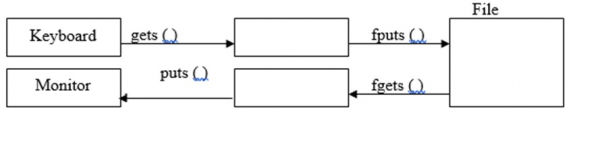# Explain fgetc() and fputc() functions in C language

CServer Side ProgrammingProgramming

File is collection of records or is a place on hard disk, where data is stored permanently.

## Operations on files

The operations on files in C programming language are as follows −

• Naming the file
• Opening the file
• Writing into the file
• Closing the file

## Syntax

The syntax for opening a file is as follows −

FILE *File pointer;

For example, FILE * fptr;

The syntax for naming a file is as follows −

File pointer = fopen ("File name", "mode");

For example,

fptr = fopen ("sample.txt", "r");
FILE *fp;
fp = fopen ("sample.txt", "w");

### fgets( ) and fputs( ) functions

fgets( ) is used for reading a string from a file.

The syntax for fgets() function is as follows −

fgets (string variable, No. of characters, File pointer);

For example,

FILE *fp;
char str ;
fgets (str,30,fp);

fputs( ) function is used for writing a string into a file.

The syntax for fputs() function is as follows −

fputs (string variable, file pointer);

For example,

FILE *fp;
char str;
fputs (str,fp);## Program

Following is the C program for using fgets() and fputs() functions −

Live Demo

#include <stdio.h>
int main(){
FILE *fptr = fopen("sample.txt","w");
fputs("TutorialPoints\n",fptr);
fputs("C programming\n",fptr);
fclose(fptr);
fptr = fopen("sample.txt","r");
char string;
while(fgets(string,30,fptr)!=NULL){
printf("%s",string);
}
fclose(fptr);
return 0;
}

## Output

When the above program is executed, it produces the following result −

TutorialPoints
C programming
Question & Answers